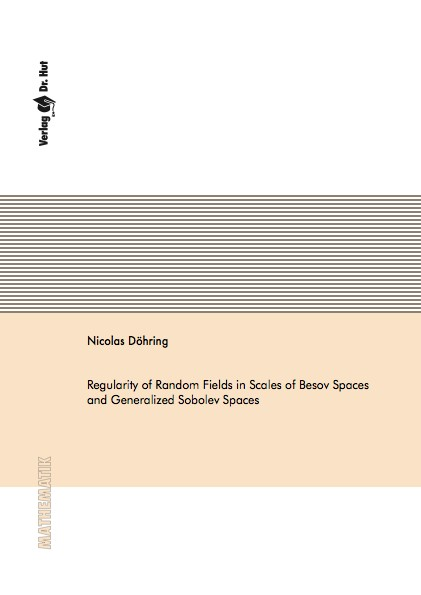Datenbestand vom 25. Mai 2020aktualisiert am 25. Mai 2020

# ISBN 9783843924368Euro 60,00 inkl. 7% MwSt

978-3-8439-2436-8, Reihe Mathematik

Nicolas Döhring
Regularity of Random Fields in Scales of Besov Spaces and Generalized Sobolev Spaces

109 Seiten, Dissertation Technische Universität Kaiserslautern (2015), Softcover, A5

## Zusammenfassung / Abstract

The main topic of this thesis is the construction of random fields whose realizations have almost surely a prescribed regularity in scales of Besov and certain Sobolev spaces. In a second step we focus on the analysis of linear and nonlinear approximation of those random fields and we establish upper and lower bounds for the corresponding approximation error. As a potential application we consider the approximation of the solution of certain stochastic partial differential equations (SPDEs).

We use wavelet characterizations for Besov and Sobolev spaces that allow to construct random fields with realizations that are almost surely contained in these spaces. We use random coefficients for a suitable basis, and study different dependence structures as well as sparsity and decay behavior of the family of random coefficients and their impact on the regularity of the realizations of the random fields.

We derive weak asymptotic bounds for the best average N-term wavelet approximation error as well as for the linear approximation error and show how they relate to the smoothness of the random field, which again can be described in terms of sparsity and decay behavior of the system of random coefficients.

The approximation rates for random fields are then used to determine approximation rates of the solution of the stochastic poisson equation, which is an example of an elliptic boundary value problem with random right-hand side. These elliptic equations have to be solved when applying an Euler scheme to a parabolic SPDE. We do that to approximate the solution of such a parabolic SPDE and we establish an upper bound for the mean square error in the case, where we can solve the elliptic subproblems exactly. We will show that we can get the same order of convergence in the case, where the subproblems have to be approximated.